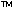DOC HOME SITE MAP MAN PAGES GNU INFO SEARCH PRINT BOOK
Floating point operations

# Extended-precision

Extended-precision floating point numbers have the following format on the Intel386microprocessor:

79 78 64 63 0
SIGN EXPONENT FRACTION
^
binary point
For extended precision, bit 63 is always a 1, and the decimal point comes after this bit. The other formats have an implicit bit 1 before the fraction, as explained in ``Normalized numbers''.

Field Position Full name
sign 79 sign bit (0==positive, 1==negative)
exponent 78-64 exponent (biased by 16,383)
fraction 63-0 fraction (bit 63=1, followed by the
decimal point. Bits 62-0 are the fraction.

Next topic: Normalized numbers
Previous topic: Double-precision